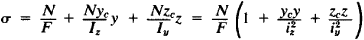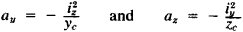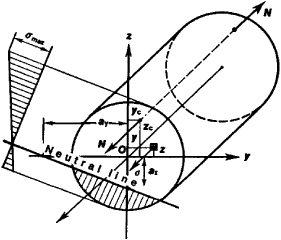# Eccentric Tension-Compression of a Rod

The following article is from The Great Soviet Encyclopedia (1979). It might be outdated or ideologically biased.

## Eccentric Tension-Compression of a Rod

(the strength of materials), deformation resulting from the action upon a rod of two equal longitudinal forces of opposite directions, parallel to the axis of the rod; a form of combined strength. Eccentric tension-compression is characterized by the composition of deflections from bending and from longitudinal forces. For eccentric tension-compression at points of a cross section with flow coordinates y and z taken with respect to the principal center of axes (see Figure 1), the normal tensions are determined according to the formulain which F is the area of the cross section, Iy and Iz are the moments of inertia of the section, iy and iz are the radii of gyration of the section, and yc and zc are the coordinates of the point of application of the longitudinal force N. The normal tensions depend linearly on the coordinates and reach maximum values at the points of the cross section farthest from the neutral line, whose position is determined by segments ay and az, cut off at the coordinate axes:If the longitudinal force is applied at the boundaries of the core of the cross section, then the neutral line either lies out-side the section or touches the profile of the section, in which case the normal stress sheet has a single value. Cases of eccentric tension-compression are often encountered in calculations for foundations, arches, and frames.Figure 1. Eccentric tension-compression of a rod

L. V. KASAB’IAN